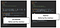Practical bayesian optimization using Goptuna

Bayesian optimization is widely used to find the global maximum or global minimum value of black-box function. Although it has been mainly studied for hyper-parameter tuning of machine learning models, it is also able to apply to any problems as long as you can define an objective function.

For example, Facebook announced Ax and Botorch. They are planning to use these softwares in wide variety of projects like following:

• Tuning Video Streaming Algorithms
• Tuning Machine Learning Models
• AR/VR Hardware Design
• Tuning Just-In-Time compiler (for HHVM)

Please watch the talk at F8 2019 for more details, Product Optimization with Adaptive Experimentation. I think that black-box optimization algorithms have a great potential for every software developers.

In this article, I investigates the existing bayesian optimization library written in Go, then introduces Goptuna which I published yesterday. It can be used for optimizing the number of goroutines of our servers written in Go and optimizing the memory buffer size of our caching systems.

Bayesian optimization library for black-box functions written in Go.
https://github.com/c-bata/goptuna

Introduction of Bayesian Optimization

First, I will explain what bayesian optimization algorithms are doing. When we have a something system and its performance depends on something continuous parameters in the range of 0 to 10. I would like to specify an optimal parameter that gives the best performance of our system.

Naive approaches like grid search and random search require a lot of evaluations to get to an optimal parameter. But we may imagine promising parameters from the past results. There may be even better evaluation values near those values that have had good results in the past. And there may be better evaluation values at the area we don’t still evaluate it. By using bayesian optimization algorithms, we can sample parameter values like human.

There are 3 state-of-the-art bayesian optimization methods:

GP (Gaussian Process) based algorithms (ex: GP-EI)

TPE (Tree of Parzen Estimators)

SMAC (Sequential Model-based Algorithm Configuration)

Investigating existing Bayesian Optimization library for Go

I googled existing libraries for bayesian optimization in Go, and I found go-bayesopt, gaussian process based bayesian optimization library. I prepared the minimization problem like following.

f(x1, x2) = (x1–1)²+ (x2–2)²

The optimal parameter of above function is (x1, x2) = (1, 2). I prepared the following program using go-bayesopt to optimize the function.

After 10 parameters are selected by random search, 90 parameters are selected by GP-UCB (GP is a surrogate model and UCB is an acquisition function). The execution results are as follows.

\$ go run _examples/main.go
...
2019/07/25 15:23:23 x: map[bayesopt.Param]float64{bayesopt.UniformParam{Name:"X1", Max:10, Min:-10}:1.0221672603083967, bayesopt.UniformParam{Name:"X2", Max:10, Min:-10}:1.8540991095989217}
2019/07/25 15:23:23 y: 0.021778

The objective function is called 100 times, then I got the f (1.022, 1.854) = 0.021778 as the best evaluation value. Looking at the following graph, you can see that go-bayesopt focuses on the points that are likely to be high evaluation value.

It works fine. But it is probably implemented for study use, so there are not enough functionalities. It takes 5 minutes for 100 evaluations because inferences from GP regression models requires the computation of inverse matrices, and generally the computation time tends to be long. It require O(t³), t is an iteration count. And if you want to use another acquisition function such as EI (Expected Improvement), you need to implement by yourself.

The Python library uses LAPACK through numpy and scipy, but in Go we want to avoid to use cgo for keeping portability. To apply wide variety of projects, it is better to be implemented in pure Go.

TPE bayesian optimization using Goptuna

TPE(Tree-structured Parzen Estimator) requires O(t), t is an iteration count. So I googled exiting TPE implementation in Go, but it’s not found. So I implemented it and published Goptuna yesterday.

As the name implies, it is a golang port of Optuna. Goptuna adopts the Define-by-Run interface like Optuna. So we can use it intuitively as follows.See the paper of Optuna at KDD 2019 for more details about Define-by-Run interface. Takuya Akiba, Shotaro Sano, Toshihiko Yanase, Takeru Ohta, Masanori Koyama. 2019. Optuna: A Next-generation Hyperparameter Optimization Framework. In The 25th ACM SIGKDD Conference on Knowledge Discovery and Data Mining (KDD ’19), August 4–8, 2019.

The results are below:

Random Search with 100 evaluations

\$ go run _examples/simple_random_search/main.go
...
Result:
- best value 2.5904889254208054
- best param map[x1:0.4405659427118902 x2:0.7883530201915825]

TPE with 100 evaluations (including 10 evaluations of random search)

\$ go run _examples/simple_tpe/main.go
...
Result:
- best value 0.6195459685895217
- best param map[x1:0.7770961438621743 x2:1.2451093857329996]

In this case, the result of TPE is better than Random Search and worse than go-bayesopt. The search spaces of objective function is low-dimensional (2-dimentions) and it doesn’t include categorical variables. Eggensperger et al. confirmed that gaussian process based bayesian optimization performs better than TPE and SMAC in low-dimensional continuous search spaces (See Towards an Empirical Foundation for Assessing Bayesian Optimization of Hyperparameters.). So in a case of high-dimensional and includes categorical parameters, I think Goptuna performs better.

Next, let’s compare the execution time. Since the objective function is a simple one that consumes little time, the time taken for this execution is dominated by the time consumed by the black box optimization library.